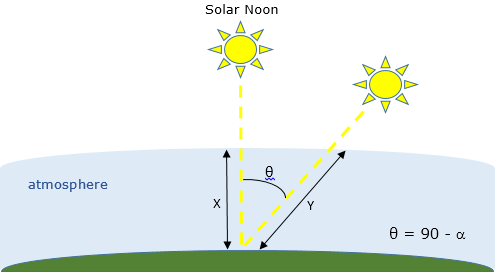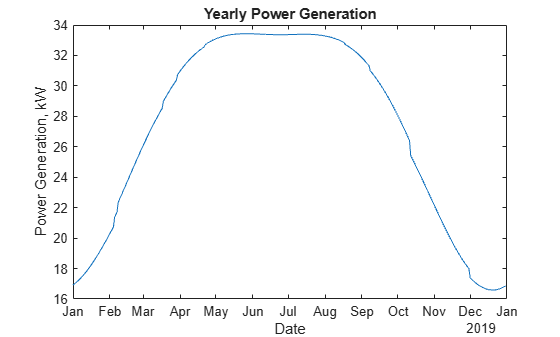Documentation

### This is machine translation

Mouseover text to see original. Click the button below to return to the English version of the page.

## Create an Interactive Narrative with the Live Editor

The following is an example of how to use the Live Editor to create an interactive narrative. Create an interactive narrative to tell a story with computations that you have used to solve a problem. This example shows how to:

• Use formatted text to describe your approach.

• Show output together with your MATLAB code.

• Use equations to describe the underlying mathematics.

• Include images to illustrate important points.

• Provide hyperlinks to background material.

• Let the reader modify parameters and re-run the analysis.

• Include plots for visualization.

• Invite colleagues to extend your analysis.

### The Overall Approach

In the Live Editor, you can include formatted text as part of the interactive narrative. Use bold, italic, and underlined text to highlight important words or ideas. Use bullets or numbers to format lists.

In this example we will estimate the power output from a typical solar panel installation. We will use 12 noon on June 1st in Boston to illustrate how to calculate the following:

• Solar time

• Solar declination and solar elevation

• Air mass and the solar radiation reaching the earth's surface

• Radiation on a solar panel given its position, tilt, and efficiency

• Power generated in a day and over the entire year

We will use these formulas to plot solar and panel radiation for our example day, and then plot the expected panel power generation over the course of a year. We'll use two MATLAB functions created for this analysis, solarCorrection and panelRadiation, to streamline the analysis.

### Solar Time

Show output together with the code that produced it. To run a section of code, go to the Live Editor tab and click the Run Section button.

Power generation in a solar panel depends on how much solar radiation reaches the panel which in turn depends on the sun's position relative to the panel as the sun moves across the sky.

lambda = -71.06; % longitude phi = 42.36; % latitude UTCoff = '-5'; % UTC offset TZ = ['UTC' UTCoff]; january1 = datetime(2016,1,1,'TimeZone',TZ); % January 1st localTime = datetime(2016,6,1,12,0,0,'TimeZone',TZ) % Noon on June 1
localTime = datetime 01-Jun-2016 12:00:00 

To calculate the sun's position for a given date and time we need to use solar time. Twelve noon solar time is defined to be the time when the sun is highest in the sky. To calculate solar time, we apply a correction to local time. That correction has two parts:

• A term which corrects for the difference between the observer's location and the local meridian

• An orbital term related to the eccentricity of the earth's orbit and its axial tilt

We'll use a MATLAB function created for this analysis called solarCorrection. For example, at noon on June 1, the solar time would be:

d = caldays(between(january1,localTime,'Day')); % Day of year solarCorr = solarCorrection(d,lambda,str2double(UTCoff)); % Correction to local time solarTime = localTime + minutes(solarCorr)
solarTime = datetime 01-Jun-2016 12:18:06 

### Solar Declination and Elevation

Include equations to describe the underlying mathematics. Create equations using LaTeX commands. To add a new equation, go to the Live Editor tab and click the Equation button. Double-click an equation to edit it in the Equation Editor.

The sun's declination ($\delta$) is the angle of the sun relative to the earth's equatorial plane. The solar declination is ${0}^{\circ }$ at the vernal and autumnal equinoxes and rises to a maximum of$23.4{5}^{\circ }$ at the summer solstice. On any given day of the year (d), declination can be calculated from the following formula:

$\delta ={\mathrm{sin}}^{-1}\left(\mathrm{sin}\left(23.45\right)\mathrm{sin}\left(\frac{360}{365}\left(d-81\right)\right)\right)$

From the declination ($\delta$) and the latitude ($\varphi$) we can calculate the sun's elevation ($\alpha$) at the current time.

$\alpha ={\mathrm{sin}}^{-1}\left(\mathrm{sin}\delta \mathrm{sin}\varphi +\mathrm{cos}\delta \mathrm{cos}\varphi \mathrm{cos}\omega \right)$

Here $\omega$ is the hour angle, which is the number of degrees of rotation of the earth between the current solar time and solar noon.

delta = asind(sind(23.45)*sind(360*(d - 81)/365)); % Declination omega = 15*(solarTime.Hour + solarTime.Minute/60 - 12); % Hour angle alpha = asind(sind(delta)*sind(phi) + ... % Elevation cosd(delta)*cosd(phi)*cosd(omega)); disp(['Solar Declination = ' num2str(delta) ' Solar Elevation = ' num2str(alpha)])
Solar Declination = 21.9634 Solar Elevation = 69.2589 

Knowing the sun's declination and the local latitude allows us to calculate the time of sunrise and sunset. Sunrise and sunset are calculated in Standard Time.

$sunrise=12-\frac{{\mathrm{cos}}^{-1}\left(-\mathrm{tan}\varphi \mathrm{tan}\delta \right)}{1{5}^{\circ }}-\frac{TC}{60}\phantom{\rule{2em}{0ex}}\phantom{\rule{2em}{0ex}}sunset=12+\frac{{\mathrm{cos}}^{-1}\left(-\mathrm{tan}\varphi \mathrm{tan}\delta \right)}{1{5}^{\circ }}-\frac{TC}{60}$

midnight = dateshift(localTime,'start','day'); sr = 12 - acosd(-tand(phi)*tand(delta))/15 - solarCorr/60; sunrise = timeofday(midnight + hours(sr)); ss = 12 + acosd(-tand(phi)*tand(delta))/15 - solarCorr/60; sunset = timeofday(midnight + hours(ss)); disp(['Sunrise = ' datestr(sunrise,'HH:MM:SS') ' Sunset = ' datestr(sunset,'HH:MM:ss') ])
Sunrise = 04:15:34 Sunset = 19:08:11 

### Air Mass and Solar Radiation

Include images to illustrate important points in your story. To include an image from a file, copy and paste an image from another source or go to the Live Editor tab and click the Image button.

As light from the sun passes through the earth's atmosphere, some of the solar radiation will be absorbed. The air mass is a function of solar elevation ($\alpha$). As shown in the diagram below, it is a measure of the length of the path of light through the atmosphere (Y) relative to the shortest possible path (X) when the sun's elevation is 90${}^{\circ }$.The larger the air mass, the less radiation reaches the ground. The air mass can be calculated from the equation

$AM=\frac{1}{\mathrm{cos}\left(90-\alpha \right)+0.5057\left(6.0799+\alpha {\right)}^{-1.6364}.}$

Then the solar radiation (in Kw/m^2) reaching the ground can be calculated from the empirical equation

$sRad=1.353*0.{7}^{A{M}^{0.678}}.$

AM = 1/(cosd(90-alpha) + 0.50572*(6.07955+alpha)^-1.6354); sRad = 1.353*0.7^(AM^0.678); % kW/m^2 disp(['Air Mass = ' num2str(AM) ' Solar Radiation = ' num2str(sRad) ' kW/m^2'])
Air Mass = 1.0688 Solar Radiation = 0.93164 kW/m^2 

### Solar Radiation on Fixed Panels

Use hyperlinks to reference supporting information from other sources. To add a hyperlink, go to the Live Editor tab and click the Hyperlink button.

Panels installed with a solar tracker can move with the sun and receive 100% of the sun's radiation as the sun moves across the sky. However, most solar cell installations have panels set at a fixed azimuth and tilt. Therefore the actual radiation reaching the panel will also depend on the sun's azimuth. The solar azimuth ($\gamma$) is the compass direction of the sun's position in the sky. At solar noon in the Northern hemisphere the sun's azimuth will be 180${}^{\circ }$ (south). The solar azimuth is calculated from this equation:

gamma = acosd((sind(delta)*cosd(phi) - cosd(delta)*sind(phi)*cosd(omega))/cosd(alpha)); if (hour(solarTime) >= 12) && (omega >= 0) gamma = 360 - gamma; end disp(['Solar Azimuth = ' num2str(gamma)])
Solar Azimuth = 191.8568 

In the northern hemisphere, a typical solar panel installation would have panels oriented toward the south with a panel azimuth ($\beta$) of $18{0}^{\circ }$. At northern latitudes a typical tilt angle ($\tau$) would be 35${}^{\circ }$. For fixed panels, the panel radiation is calculated from the total solar radiation using this equation:

$pRad=sRad\left[\mathrm{cos}\left(\alpha \right)\mathrm{sin}\left(\tau \right)\mathrm{cos}\left(\beta -\gamma \right)+\mathrm{sin}\left(\alpha \right)\mathrm{cos}\left(\tau \right)\right]$

beta = 180; % Panel azimuth tau = 35; % Panel tilt pRad = sRad*max(0,(cosd(alpha)*sind(tau)*cosd(beta-gamma) + sind(alpha)*cosd(tau))); disp(['Panel Radiation = ' num2str(pRad) ' kW/m^2'])
Panel Radiation = 0.8989 kW/m^2 

### Panel Size and Efficiency

Let the reader change the values of parameters in the analysis to see how different parameter values affect the results.

So far our calculations reflect the theoretical maximum solar radiation available for power conversion. However, solar panels do not convert 100% of available solar radiation into electricity. The efficiency of a solar panel is the fraction of the radiation reaching the panel that is converted into electricity. The efficiency of a solar panel depends on the design and materials of the cell.

Typically, a residential installation includes 20 ${m}^{2}$ of solar panels with an efficiency of 25%. You can modify the parameters below to see how efficiency and size affect panel power generation.

eff = 0.36; % Panel efficiency pSize = 25; % Panel size in m^2 pElec = eff*pSize*pRad; % Panel electric output in kW disp(['Expected electical output = ' num2str(pElec) ' kW'])
Expected electical output = 8.0901 kW 

### Power Generation Over Time

Create plots in the Live Editor together with the code that produced them. Double-click a plot to open it in a separate figure window.

We can now calculate how much power a panel will generate on any day of the year. To simplify the analysis, we'll use a function called panelRadiation that uses the equations above to calculate hourly total solar radiation and panel radiation for a given date and location. The parameter isFixed is set to 1 for fixed panels, 0 for tracking panels. Try different dates to see how the solar and panel radiation changes depending on the time of year.

[time, sRad, pRad] = panelRadiation(date, longitude, latitude, UTCoff, panelTilt, panelAzimuth, isFixed)

isFixed = 1; selectedYear = 2016; selectedMonth = 1; selectedDay = 1; date = datetime(selectedYear,selectedMonth,selectedDay,'TimeZone',TZ); [times, sRad, pRad] = panelRadiation(date, lambda, phi, UTCoff, tau, beta, isFixed) ; plot(times,sRad,times,pRad) title(['Solar and Panel Radiation for ' datestr(date,'mmmm dd yyyy')]) xlabel('Hour of Day'); ylabel('Radiation, kW/m^2') legend('Available Solar Radiation','Solar Radiation on Panel', 'Location','South')We can repeat the calculation to estimate power generation for each day of the year.

date = datetime(2015,1,1:365,'TimeZone',TZ); % Create a vector of days in the year for i = 1:365 [times, sRad, pRad] = panelRadiation(date(i), lambda, phi, UTCoff, tau, beta, isFixed) ; radiation = sum(pRad(1:end-1)+pRad(2:end))/2; dailyPower(i) = eff*pSize*radiation; end plot(date,dailyPower) title('Yearly Power Generation') xlabel('Date'); ylabel('Power Generation, kW-hrs')yearlyPower = sum(dailyPower); disp(['Expected annual power output = ' num2str(yearlyPower) ' kW-hrs'])
Expected annual power output = 17917.7889 kW-hrs 

### Extending the Analysis

Share your analysis with colleagues. Invite them to reproduce or extend your analysis. Work collaboratively using the Live Editor.

In reality, true power output from a solar installation will be significantly affected by local weather conditions. An interesting extension of this analysis would be to see how cloud cover affects the results. In the US, we can use data from the following government websites.